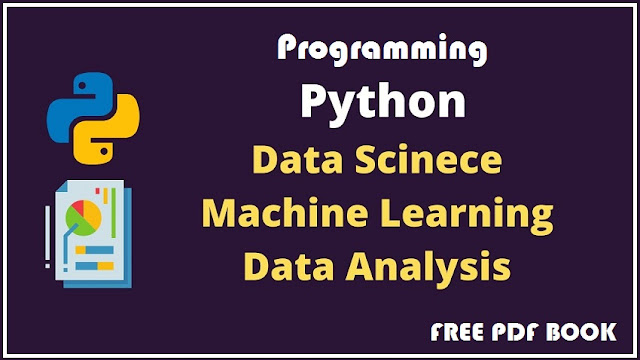# Python Programming The Crash Course for Python Projects BOOK FULL FREE 2022.

Python Programming The Crash Course for Python Projects

Learn the Secrets of Machine Learning, Data Science Analysis and Artificial Intelligence.

Introduction to Deep Learning for Beginners.

BOOK FULL FREE 2022.The different machine learning techniques with Python:

There are different algorithms, techniques and methods of ML that can be used to build models in order to solve real-life problems using data.

In this section, we will discuss these different types of methods. Supervised learning: This learning method or algorithm takes the sample of data, that is, the learning data, and the output, that is, the labels or responses, associated with each sample of data during the learning process.

The main purpose of supervised learning algorithms is to learn an association between input data samples and corresponding outputs after performing multiple instances of training data. K-Nearest Neighbors (K nearest neighbours) SVM Kernel Naïve Bayes (Bayesian networks) Classification by decision tree Random Forest Classification The KNN (K-Nearest Neighbors) algorithm is a simple, non-parametric supervised machine learning algorithm that can be used to solve classification and regression problems. We will now introduce you to the project of detecting fruit from several characteristics. Based on the value of the mass, width, height and color score, we will determine if a fruit is an apple, tangerine, orange or lemon. For example, we have x as input variable and y as output variable.

The objective of a supervised learning algorithm is to find an f function for matching the input variable (x) with the output variable (Y), that is, an expression of the type Y=f(x). In order to obtain new input data (x), we can easily predict the output variable (Y) for these new input data.